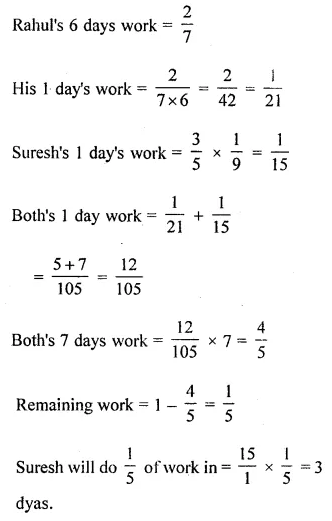# ML Aggarwal Class 8 Solutions for ICSE Maths Chapter 1 Rational Numbers Objective Type Questions

## ML Aggarwal Class 8 Solutions for ICSE Maths Chapter 1 Rational Numbers Objective Type Questions

Mental Maths

Question 1.
Fill in the blanks:
(i) The product of two rational numbers is a ……….
(ii) Subtraction of rational numbers is …….. commutative.
(iii) The rational number $$\frac { -7 }{ 4 }$$ lies ……. of zero on the number line.
(iv) Division of rational numbers is ……… associative.
(v) $$\frac { p }{ q }$$ ÷ 0 is ……..
(vi) Negative of a rational number is called its ………
(vii) Multiplicative identity of rational numbers is ……….
(viii) Multiplication of rational numbers is ……. over addition.
(ix) Division of a rational number by a non-zero rational number is a ………..
(x) The rational number which is additive inverse of itself is …………
Solution: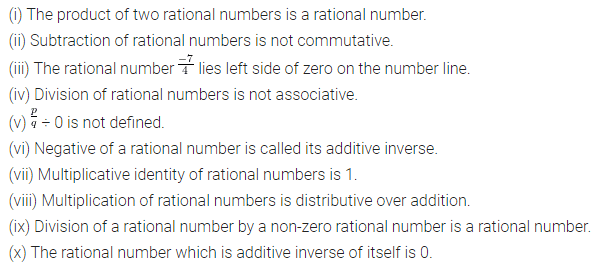Question 2.
State whether the following statements are true (T) or false (F):
(i) $$\frac { -5 }{ 9 }$$ is the additive inverse of $$\frac { 5 }{ 9 }$$.
(ii) Every integer is a rational number.
(iii) Zero has its multiplicative inverse.
(iv) Every rational number is an integer.
(v) Division of two rational numbers is alwasy closed.
(vi) Non-terminating, non-recurring decimal numbers are rational numbers.
(vii) 0 is the multiplicative identity of rational numbers.
(viii) Non-terminating recurring decimal numbers are not rational numbers.
(ix) Subtraction of two rational numbers is not associative.
(x) Reciprocal of 1 is 1.
(xi) The multiplicative inverse is also called a reciprocal.
(xii) Between two different rational numbers, there are infinitely many number of rational numbers.
Solution: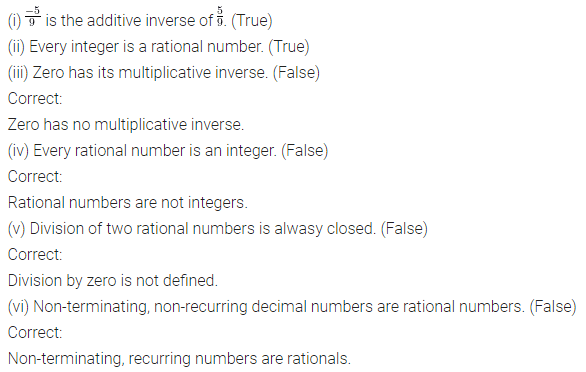Multiple Choice Questions

Choose the correct answer from the given four options (3 to 18):
Question 3.
Additive inverse of $$\frac { -2 }{ -5 }$$ is
(a) $$\frac { 2 }{ 5 }$$
(b) $$\frac { 5 }{ 2 }$$
(c) $$\frac { 2 }{ -5 }$$
(d) $$\frac { 5 }{ -2 }$$
Solution:Question 4.
Multiplicative inverse of $$\frac { -3 }{ 7 }$$ is
(a) $$\frac { 7 }{ 3 }$$
(b) $$\frac { -7 }{ 3 }$$
(c) $$\frac { 3 }{ 7 }$$
(d) None of these
Solution: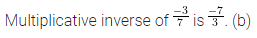Question 5.
Sum of a rational number and its additive inverse is
(a) 1
(b) 0
(c) -1
(d) None of these
Solution:Question 6.
Rational numbers are not closed under
(b) subtraction
(c) multiplication
(d) division
Solution:Question 7.
0 ÷ $$\frac { 2 }{ 3 }$$ is equal to
(a) $$\frac { 2 }{ 3 }$$
(b) $$\frac { 3 }{ 2 }$$
(c) 0
(d) not defined
Solution:Question 8.
$$\frac { 2 }{ 3 }$$ ÷ 0 is equal to
(a) $$\frac { 2 }{ 3 }$$
(b) $$\frac { 3 }{ 2 }$$
(c) 0
(d) not defined
Solution:Question 9.
$$\frac { p }{ q } +\left( \frac { r }{ s } +\frac { t }{ u } \right) =\left( \frac { p }{ q } +\frac { r }{ s } \right) +\frac { t }{ u }$$ is called
(a) commutative property
(b) associative property
(c) distributive property
(d) None of these
Solution:Question 10.
Multiplication of a non-zero rational number and its reciprocal is
(a) 0
(b) 1
(c) -1
(d) None of these
Solution:Question 11.
Product of rational number $$\frac { -2 }{ 5 }$$ and its additive inverse is
(a) 0
(b) 1
(c) $$\frac { -4 }{ 25 }$$
(d) $$\frac { -5 }{ 2 }$$
Solution: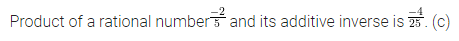Question 12.
Sum of rational number $$\frac { 4 }{ 7 }$$ and its reciprocal is
(a) $$\frac { 28 }{ 65 }$$
(b) $$\frac { 65 }{ 28 }$$
(c) $$\frac { -28 }{ 65 }$$
(d) $$\frac { -65 }{ 28 }$$
Solution:Question 13.
Sum of two rational numbers is 0, if one ofthem is $$\frac { -4 }{ 5 }$$, then other is
(a) $$\frac { 5 }{ 4 }$$
(b) $$\frac { 4}{ 5 }$$
(c) $$\frac { -5 }{ 4 }$$
(d) $$\frac { -4 }{ 5 }$$
Solution: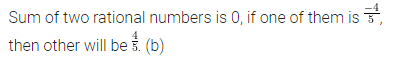Question 14.
Product of two rational numbers is 1, if one of them is $$\frac { 10 }{ 3 }$$, then other is
(a) $$\frac { 3 }{ 10 }$$
(b) $$\frac { -3}{ 10 }$$
(c) $$\frac { 10 }{ 3 }$$
(d) None of these
Solution:Question 15.
Rational number represented by the point P on the number line is(a) $$\frac { -5 }{ 7 }$$
(b) $$\frac { -3}{ 7 }$$
(c) $$\frac { -5 }{ 8 }$$
(d) $$\frac { -4 }{ 8 }$$
Solution: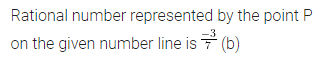Question 16.
What should be subtracted from $$\frac { -5 }{ 3 }$$ to get $$\frac { -2 }{ 7 }$$?
(a) $$\frac { 29 }{ 21 }$$
(b) $$\frac { -21}{ 29 }$$
(c) $$\frac { -29 }{ 21 }$$
(d) $$\frac { 21 }{ 29 }$$
Solution: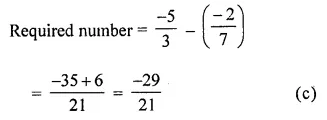Question 17.
Reciprocal of a negative number is
(a) positive
(b) negative
(c) can not say
(d) does not exist
Solution: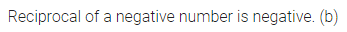Question 18.
Which of the following statement is true?Solution:Value Based Questions

Question 1.
Ram donated $$\frac { 1 }{ 10 }$$ of his salary to an orphanage, $$\frac { 1 }{ 3 }$$ of his salary spent on food, $$\frac { 1 }{ 4 }$$ of salary on rent and electricity and $$\frac { 1 }{ 20 }$$ of his salary on telephone. This month he donated ₹ 5000 in Prime Minister relief fund for Uttarakhand victims. He was left with ₹ 3000 with him, find his monthly salary. Should we donate the money for needy people? What values are being promoted?
Solution:Question 2.
In an Examination $$\frac { 1 }{ 3 }$$ of the total students used unfair means and out of which $$\frac { 1 }{ 4 }$$ caught red handed while cheating. If 5 students caught red handed then find the total number of students appeared in exam.
Why should we not use unfair means in an examination?
What values are being promoted?
Solution: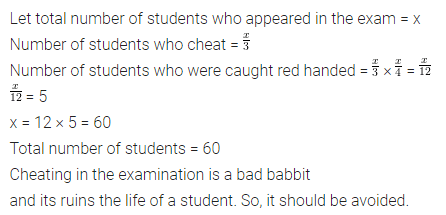Higher Order Thinking Skills (HOTS)

Question 1.
Area ol a square is 4 sq. in more than $$\frac { 2 }{ 3 }$$ of the area of a rectangle. If the area of square is 64 sq. m, then find the dimensions of the rectangle, given that breadth is $$\frac { 2 }{ 5 }$$ of length.
Solution: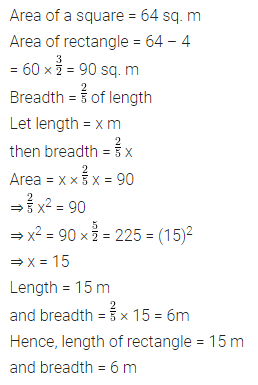Question 2.
Rahul can do $$\frac { 2 }{ 7 }$$ of a certain work in 6 days while Suresh can do $$\frac { 3 }{ 5 }$$ of the same work in 9 days. They started work together but after 7 days Rahul left the work. Find in how many days Suresh can complete the remaining work?
Solution: